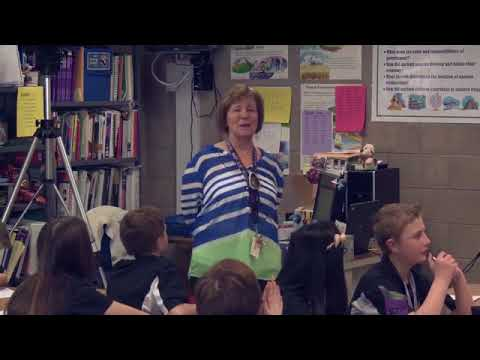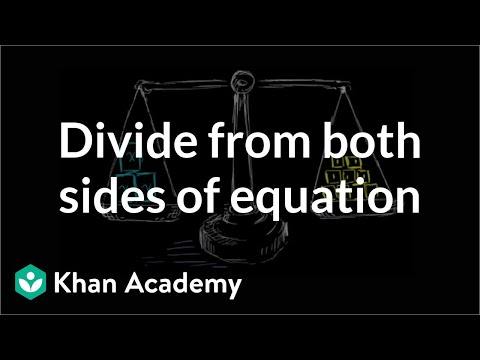Video

# One-step multiplication & division equations: fractions & decimals (Full video)

Khan Academy

## Supporting grades: 6

Description: Learn how to solve equations in one step by multiplying or dividing a number from both sides. These problems involve decimals and fractions. Let's get some practice solving some equations, and we're gonna set up some equations that are a little bit hairier than normal, they're gonna have some decimals and fractions in them. So let's say I had the equation 1.2 times c is equal to 0.6. So one thing I like to do is say okay, I have the c on the left hand side, and I'm just multiplying it by 1.2, it would be great if this just said c. Well I could just divide by 1.2 but as we've seen multiple times, you can't just do that to the left hand side, that would change, you no longer could say that this is equal to that if you only operate on one side.

You must log inorsign upif you want to:*

*Teacher Advisor is 100% free.

### Other videos you might be interested in### Using Substitution to Understand Equations and Inequalities (Full video)

#### Student Achievement Partners